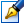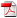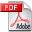##Mathematical Modeling of nonlinear processes in semiconductor device

 Author: Sprincean Galina Degree: doctor of physics and mathematics Speciality: 01.01.09 - Mathematical cybernetics and operation research Year: 2022 Scientific adviser: Vladimir Paţiucdoctor, associate professor (docent), Institute of Applied Physics of the Institution: Moldova State University

### Status

The thesis was presented on the 20 May, 2022 at the meeting of the Scientific Council and now it is under consideration of the National Council.

### Abstract1.84 Mb / in romanian

### Thesis

CZU 519.6 (043.3)3.62 Mb / in romanian
0 pages

### Keywords

Nonlinear processes, semiconductor devices, mathematical modeling, finite difference schemes, Scharfetter-Gummel scheme, Gummel's algorithm, Newton's method, iterative methods, semi-iterative methods, variational methods

### Summary

Thesis structure: The thesis is written in Romanian and contains introduction, four chapters, general conclusions and recommendations, bibliography of 113 titles, 3 annexes, 126 pages with basic text, 4 figures, 16 tables. The results are published in 14 scientific papers.

Purpose and objectives of the work: Elaboration and theoretical argumentation of numerical algorithms for mathematical modeling of nonlinear processes in a semiconductor device, with the identification of the most efficient ones. And as objectives: establishing the framework of numerical modeling, elaboration of the conceptual model, using appropriate mathematical or graphical notations, realization of the mathematical model, verification and validation of elaborated numerical algorithms, performing numerical experiments generating results, analysis and interpretation of results.

Results obtained: The problem that mathematically models nonlinear processes in the semiconductor device has been solved. Result that leads to the extension of the area of solvable nonlinear problems, being completed with algorithms for solving systems with partial, nonlinear derivatives and with two types of boundary conditions that act on disjoint portions. The area of application of these results is wide both theoretically (developing even more efficient algorithms that mathematically model nonlinear processes in semiconductor devices) and practically (with the implementation of the algorithms developed in the research by creating the appropriate software, which can be used for real modeling of semiconductor devices).

Scientific novelty and originality: The problem of mathematical modeling of nonlinear processes has been and remains one of the most difficult problems of semiconductor physics, on the grounds that nonlinear processes are uncontrollable. In the thesis, the efficient numerical algorithms that mathematically model nonlinear processes in a semiconductor device, which involved solving a system of equations with partial, nonlinear derivatives and two types of boundary conditions acting on disjoint portions, have been elaborated and theoretically argued.

Theoretical significance and applicative value of the work: Extending the area of research and application of mathematical modeling to solving nonlinear problems, the case of the semiconductor device. The results obtained can be used as support for an optional master's course.

Implementation of scientific results: The scientific results will be used in the training process at the Faculties of Mathematics and Computer Science, Physics and Engineering of the State University of Moldova, within the teaching of the specialization disciplines. The developed algorithms were developed in the form of MatLab programs.